All the roads of a city are either perpendicular or parallel to one another. The roads are all straight. Roads A, B, C, D and E are parallel to one another. Roads G, H, I, J, K, L and M are parallel to one another.

Which is necessarily true ?

###### Option:
A. E and B intersect.
B. D is 2 km west of B.
C. D is at least 2 km west of A.
D. M is 1.5 km north of L.
E. I is 1 km north of L.
Answer: D . M is 1.5 km north of L.

Justification:

Clearly from statements (1) and (2), figure 1 follows; from statement (3), figure 2 follows; from statement (4), figure 3 follows; and from statement (5), figure 4 follows; and from statements (6) and (7), figure 5 follows.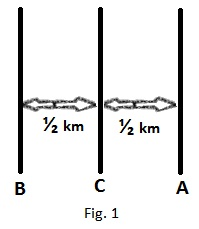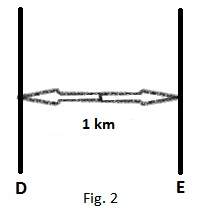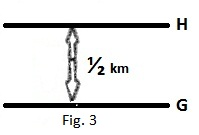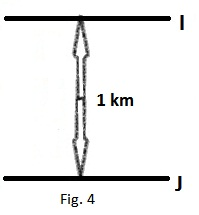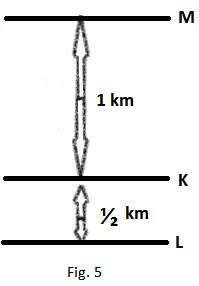It follows from figure 5 that distance of M from L = LK + KM = ½ + 1 = 1.5 km and M is to the north of L.